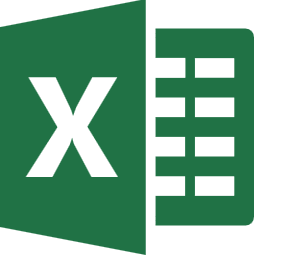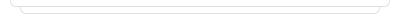Free Excel Training and Courses### First Course

Making a simple budget by using basic functions.### First Course

Making a simple budget by using basic functions.### Travel Budget

Create a "Travel Budget" sample.### Travel Budget

Create a "Travel Budget" sample.### Practice: Basic functions-III

Using basic functions with more practices.### Practice: Basic functions-III

Using basic functions with more practices.### Profitability of a Theater

Using four operations and statistical functions over a scenario about play tickets status of a theater.### Practice: Basic functions-IV

Using basic functions with more practices.### Practice: Basic functions-IV

Using basic functions with more practices.### Practice: Working with operators

Using basic functions with more practices.### Practice: Working with operators

Using basic functions with more practices.### First Course

Making a simple budget by using basic functions.### Travel Budget

Create a "Travel Budget" sample.### Practice: Basic functions-III

Using basic functions with more practices.### Practice: Basic functions-IV

Using basic functions with more practices.### Practice: Working with operators

Using basic functions with more practices.